### Home > INT3 > Chapter 10 > Lesson 10.1.4 > Problem10-57

10-57.
1. Solve for x in each of the following triangles. What methods can be used for calculating unknown parts of triangles? Homework Help ✎

1.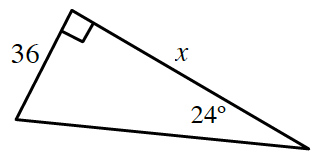2.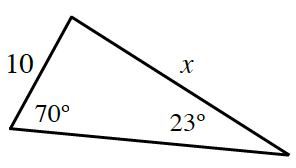3.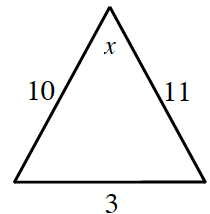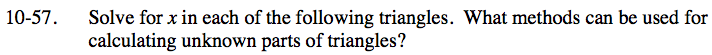What laws and theorems relate the angles of a triangle to the sides?
Or relate the lengths of the sides of a triangle to each other?
Use them to help you find the unknown parts of these triangles.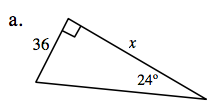Which trigonometric ratio relates the side opposite the angle to the side adjacent to it?

x ≈ 80.86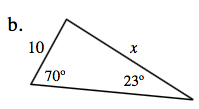Use the Law of Sines.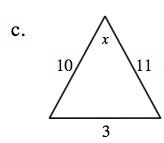Use the Law of Cosines.

x ≈ 15.50°